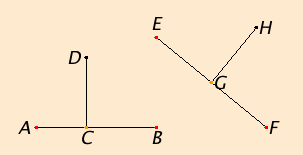# Postulate 4

That all right angles equal one another.

## GuideIn the definition of right angle, it is clear that the two angles at the foot of a perpendicular, such as angles ACD and BCD, are equal. This postulate says that an angle at the foot of one perpendicular, such as angle ACD, equals an angle at the foot of any other perpendicular, such as angle EGH.

This postulate forms the basis of angle measurement. The only angle measurement that occurs in the Elements is in terms of right angles. For instance, in proposition I.17 the sum of two angles is shown to be less than two right angles. Another example is in the proof of proposition II.9 in which two angles are shown to each be half of a right angle, so they are equal. Also, in proposition III.16 this postulate is used to claim that two angles cannot sum to less than two right angles yet be equal to two other right angles.

This postulate is first explicitly used in proposition I.14.